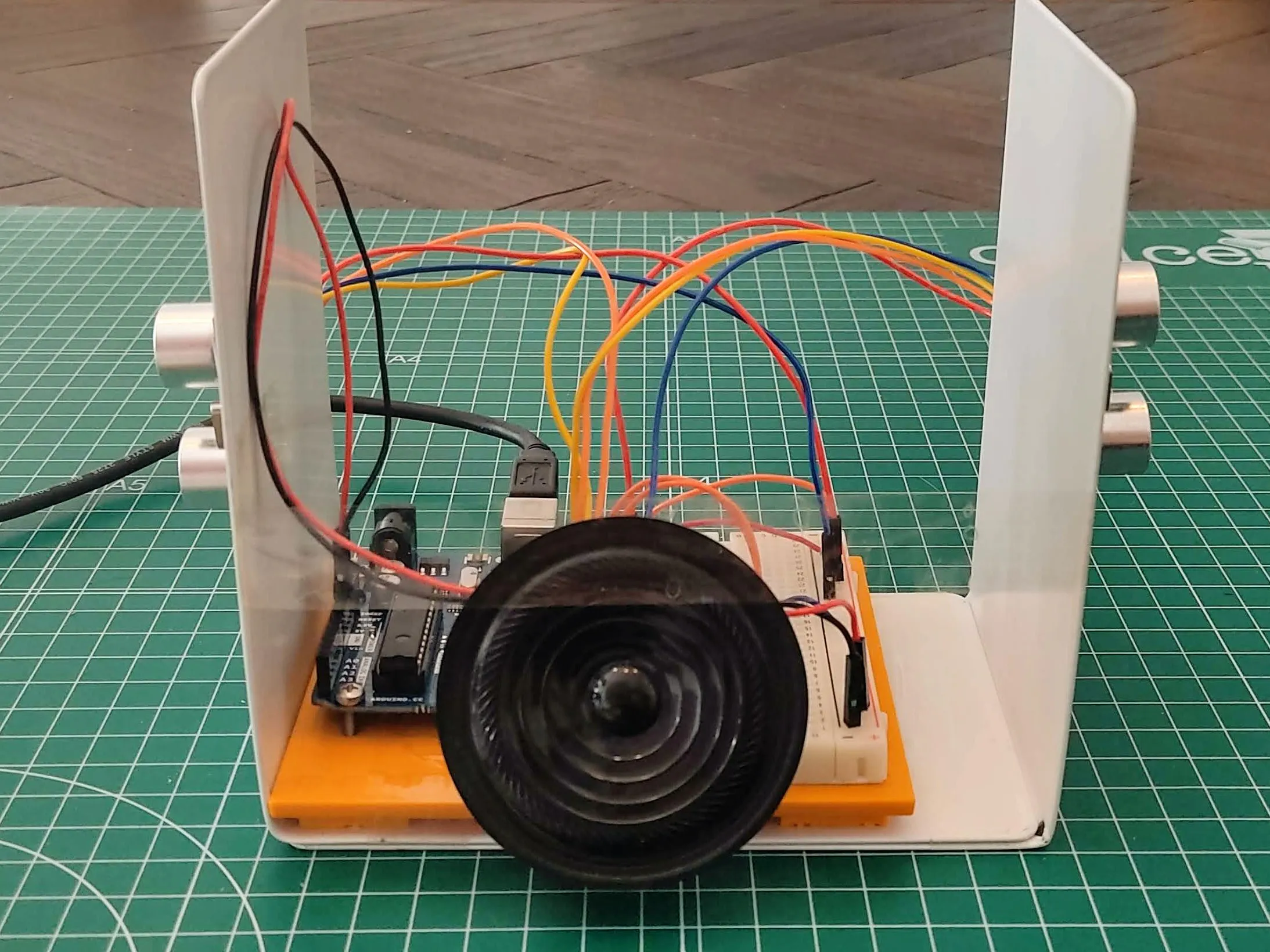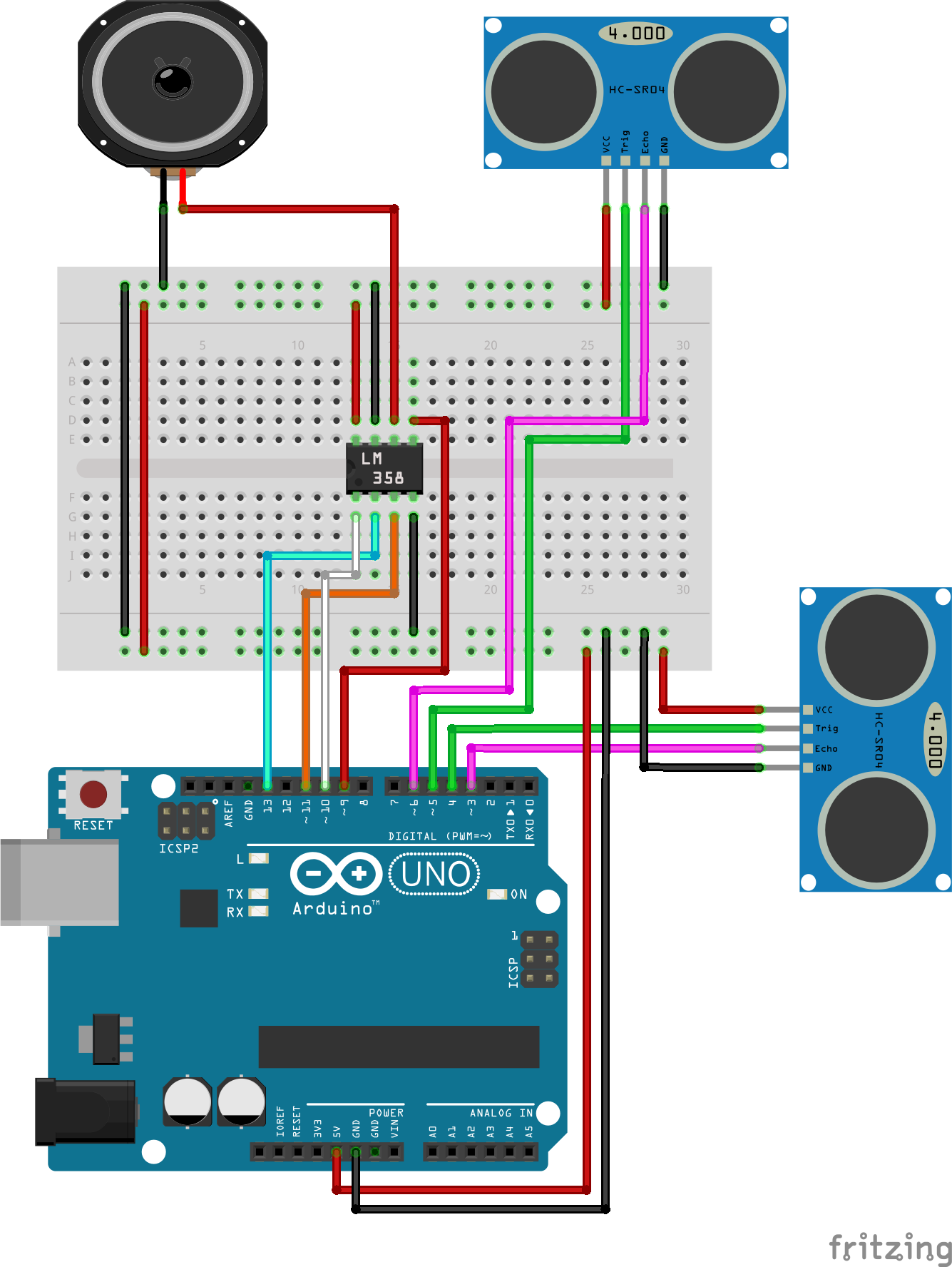Project tutorialTheremino is a theremin built with an Arduino UNO and two ultrasound sensors.

• 2,741 views
• 1 comment
• 5 respects

## Components and supplies

### What is a theremin?

A theremin is an electronic musical instrument controlled without physical contact by the thereminist (performer). It was patented by Leon Theremin in 1928. The instrument's controlling section usually consists of two metal antennas that sense the relative position of the thereminist's hands and control oscillators for frequency with one hand, and amplitude (volume) with the other [Wikipedia]. You might have already heard to theremin’s music in 1950's SciFi movies or the Beach Boys' song Good Vibrations.

In this project we are going to sort of emulate a theremin using Arduino. Most of arduino theremin tutorials use only one sensor, usualy a photosensor, few of them an ultrasonic sensor. In this one we want to use two ultrasonic sensors so we can control both frequency and amplitude, which is closer to how the original works. We will use thetwo sensors to detect distances that will be used in a function to translate them to tone and volume.

### The sensors

Generic ultrasonic sensors have four pins:

• Voltage input.
• Voltage ground.
• Trigger: Sends an ultrasonic pulse through a digital write on the program.
• Echo: Receives the ultrasonic pulse when it bounces back.

The time it takes the pulse from being sent to get back to the board is read and stored in a variable. We will convert this data to distance units (cm).

### Volume (amplitude)

To control the amplitude we use the NewPing library and the sonar.ping_cm function which sends a ping, returning the distance in centimeters or 0 (zero) if no ping echo within the set distance limit. This information is stored in the variable “distance”. The distance limit is set to 35 (cm) but you can change this based on what you feel is better for you.

### Tone (frequency)

To convert each distance to a different frequency we are using the NewTone library. We're using the equal temperament system to calculate 12 musical notes. We store this distance in the variable “distance2”, that is used to calculate a range of about 50-360 Hz dividing it by 12. In summary, for every different value of distance we have a different frequency musical note. Sort of.

### Sound (output)

A regular loudspeaker is connected directly to the output pins of the digital potentiometer. The different values of resistance will be perceived as volume variations. You might want to use an amplifier but is not really necessary.

## Code

##### Arduino Theremin codeArduino
```/*
This projects is a theremin created with two ultrasonic sensors, one controls the frequency (tone) and the other controls the amplitude (volume).
This project is a fork of two projects:
- https://create.arduino.cc/projecthub/opus9/theremino-f72d32 (this project had library conflicts)
- https://create.arduino.cc/projecthub/jvmccall/ultrasonic-theremin-033d6f (this project had typo errors in the code and a mistake in the schema)
Resource on controlling similar potentiometer using SPI protocol, provides example code and electrical setup: http://www.learningaboutelectronics.com/Articles/MCP4131-digital-potentiometer-circuit.php
*/

/*
Potentiometer conecctions:
1 - Arduino pin ~9
2 - Speaker +
3 - GND
4 - 5V
5 - Arduino pin ~10
6 - Arduino pin 13
7 - Arduino pin ~11
8 - GND
*/

#include <NewTone.h>
#include <NewPing.h>
#include <SPI.h>

#define MIN_DISTANCE 35

NewPing sonar(5, 6, 35); // the first and the second number are the pins of the sensor of volume, the third is the maximum distance

// For SPI
int CS= 10;

int echo = 3;
int trigger = 4;

int distance;
int PotValue;

unsigned long TimeResponse;
float distance2;
float tone1;

void setup() {
// set all the pins
Serial.begin(9600);
pinMode(trigger, OUTPUT); // tone sensor
pinMode(echo, INPUT); // tone sensor
pinMode (CS, OUTPUT);
SPI.begin();

// this has the speaker emit a start up sound from Low to High to Low
digitalPotWrite(255);

for (int i = 50; i <= 400; i++)
{
pinMode(9, OUTPUT);
NewTone(9,i);
delay(10);
}

delay(500);

for (int i = 400; i >= 50; i--)
{
pinMode(9, OUTPUT);
NewTone(9,i);
delay(10);
}
}

void loop() {
digitalWrite(trigger, HIGH);
delayMicroseconds(10);   // creates a 10 microsecond pulse
digitalWrite(trigger, LOW);
TimeResponse = pulseIn(echo, HIGH);  // records how long it took to receive echo of the pulse
distance2 = TimeResponse/58;  // calculates distance in centimeters

if (distance2 < MIN_DISTANCE) {
// conversion of distance into a value for a sound
tone1 = 50.0*pow(2,(distance2/12.0));  // calculate frequency, range of about 50-360 Hz
pinMode(9, OUTPUT);
NewTone(9,tone1);
} else {
pinMode(9, OUTPUT); // this sets the sound to a high pitched noise when no distance is detected
NewTone(9,0);
}

distance = sonar.ping_cm(); // uses the library NewPing to calculate distance

// distance is converted to Potentiometer relevant values
int PotValue = map(distance, 0, 35, 240, 255); // only use a range of resistance which is very low otherwise the speaker is too quiet because it is only a 8 ohm speaker

if (distance == 0) // sets volume to max when no hand is detected or if hand is too far away
{
PotValue = 255;
}

digitalPotWrite(PotValue);
delay(10);
}

int digitalPotWrite(int value) {
digitalWrite(CS, LOW); // this uses SPI protocol to communicate with the potentiometer and sets the resistance
SPI.transfer(value);
digitalWrite(CS, HIGH);
}
```

## Schematics#### Team members

• Tutorial in which this one is based by jvmccall
• Tutorial in which is based the tutorial that this one is based o_o' by opus9

July 23, 2021

#### Members who respect this project

See similar projects
you might like

#### Theremino

Project showcase by opus9

• 10,499 views
• 16 respects

#### Ultrasonic Theremin

Project showcase by jvmccall

• 3,860 views
• 5 respects

#### Arduino Theremin with A Minor Pentatonic

Project tutorial by pollux labs

• 4,837 views
• 1 comment
• 14 respects

#### Real Theremin Using Open.Theremin Shield for Arduino

Project showcase by Urs Gaudenz

• 30,928 views
• 34 respects

#### Ultrasonic Theremin

Project tutorial by squrril

• 5,002 views